Courses

# General Introduction (Part - 1) - Energy Methods in Structural Analysis Civil Engineering (CE) Notes | EduRev

## Civil Engineering (CE) : General Introduction (Part - 1) - Energy Methods in Structural Analysis Civil Engineering (CE) Notes | EduRev

The document General Introduction (Part - 1) - Energy Methods in Structural Analysis Civil Engineering (CE) Notes | EduRev is a part of the Civil Engineering (CE) Course Structural Analysis.
All you need of Civil Engineering (CE) at this link: Civil Engineering (CE)

Instructional Objectives

After reading this you will be able to

1. Differentiate between various structural forms such as beams, plane truss, space truss, plane frame, space frame, arches, cables, plates and shells.
2. State and use conditions of static equilibrium.
3. Calculate the degree of static and kinematic indeterminacy of a given structure such as beams, truss and frames.
4. Differentiate between stable and unstable structure.
5. Define flexibility and stiffness coefficients.
6. Write force-displacement relations for simple structure.

Introduction

Structural analysis and design is a very old art and is known to human beings since early civilizations. The Pyramids constructed by Egyptians around 2000 B.C. stands today as the testimony to the skills of master builders of that civilization. Many early civilizations produced great builders, skilled craftsmen who constructed magnificent buildings such as the Parthenon at Athens (2500 years old), the great Stupa at Sanchi (2000 years old), Taj Mahal (350 years old), Eiffel Tower (120 years old) and many more buildings around the world. These monuments tell us about the great feats accomplished by these craftsmen in analysis, design and construction of large structures. Today we see around us countless houses, bridges, fly-overs, high-rise buildings and spacious shopping malls. Planning, analysis and construction of these buildings is a science by itself. The main purpose of any structure is to support the loads coming on it by properly transferring them to the foundation. Even animals and trees could be treated as structures. Indeed biomechanics is a branch of mechanics, which concerns with the working of skeleton and muscular structures. In the early periods houses were constructed along the riverbanks using the locally available material. They were designed to withstand rain and moderate wind. Today structures are designed to withstand earthquakes, tsunamis, cyclones and blast loadings. Aircraft structures are designed for more complex aerodynamic loadings. These have been made possible with the advances in structural engineering and a revolution in electronic computation in the past 50 years. The construction material industry has also undergone a revolution in the last four decades resulting in new materials having more strength and stiffness than the traditional construction material.

In this book we are mainly concerned with the analysis of framed structures (beam, plane truss, space truss, plane frame, space frame and grid), arches, cables and suspension bridges subjected to static loads only. The methods that we would be presenting in this course for analysis of structure were developed based on certain energy principles, which would be discussed in the first module.

Classification of Structures

All structural forms used for load transfer from one point to another are 3- dimensional in nature. In principle one could model them as 3-dimensional elastic structure and obtain solutions (response of structures to loads) by solving the associated partial differential equations. In due course of time, you will appreciate the difficulty associated with the 3-dimensional analysis. Also, in many of the structures, one or two dimensions are smaller than other dimensions. This geometrical feature can be exploited from the analysis point of view. The dimensional reduction will greatly reduce the complexity of associated governing equations from 3 to 2 or even to one dimension. This is indeed at a cost. This reduction is achieved by making certain assumptions (like Bernoulli-Euler’ kinematic assumption in the case of beam theory) based on its observed behaviour under loads. Structures may be classified as 3-, 2- and 1-dimensional (see Fig. 1.1(a) and (b)). This simplification will yield results of reasonable and acceptable accuracy. Most commonly used structural forms for load transfer are: beams, plane truss, space truss, plane frame, space frame, arches, cables, plates and shells. Each one of these structural arrangement supports load in a specific way.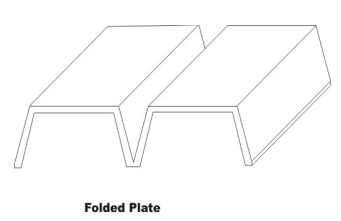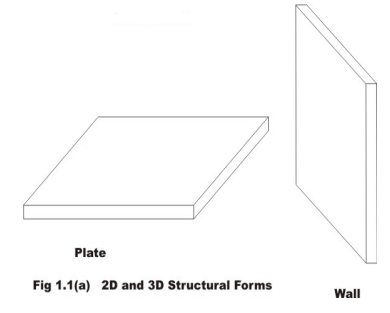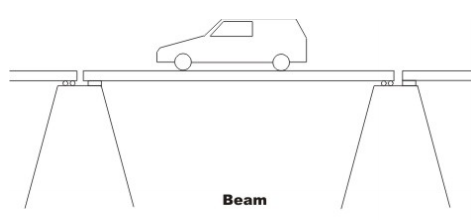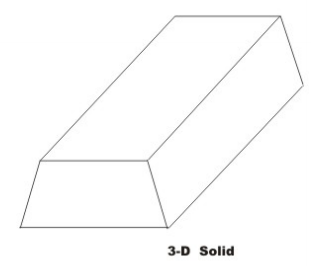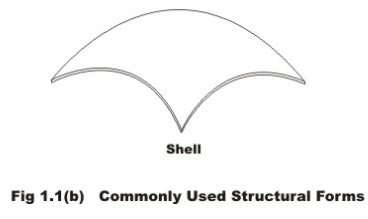Beams are the simplest structural elements that are used extensively to support loads. They may be straight or curved ones. For example, the one shown in Fig. 1.2 (a) is hinged at the left support and is supported on roller at the right end. Usually, the loads are assumed to act on the beam in a plane containing the axis of symmetry of the cross section and the beam axis. The beams may be supported on two or more supports as shown in Fig. 1.2(b). The beams may be curved in plan as shown in Fig. 1.2(c). Beams carry loads by deflecting in the same plane and it does not twist. It is possible for the beam to have no axis of symmetry. In such cases, one needs to consider unsymmetrical bending of beams. In general, the internal stresses at any cross section of the beam are: bending moment, shear force and axial force.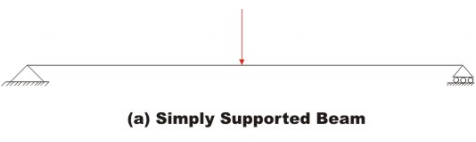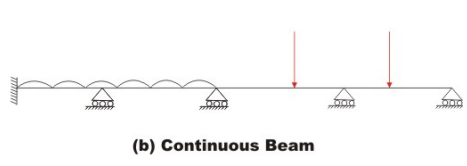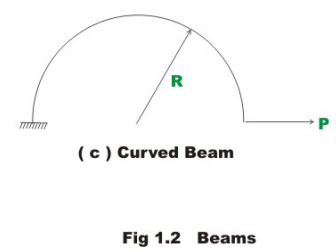In India, one could see plane trusses (vide Fig. 1.3 (a),(b),(c)) commonly in Railway bridges, at railway stations, and factories. Plane trusses are made of short thin members interconnected at hinges into triangulated patterns. For the purpose of analysis statically equivalent loads are applied at joints. From the above definition of truss, it is clear that the members are subjected to only axial forces and they are constant along their length. Also, the truss can have only hinged and roller supports. In field, usually joints are constructed as rigid by welding. However, analyses were carried out as though they were pinned. This is justified as the bending moments introduced due to joint rigidity in trusses are negligible. Truss joint could move either horizontally or vertically or combination of them. In space truss (Fig. 1.3 (d)), members may be oriented in any direction. However, members are subjected to only tensile or compressive stresses. Crane is an example of space truss.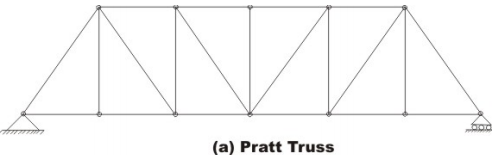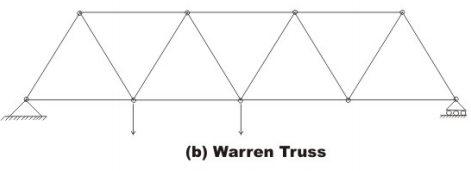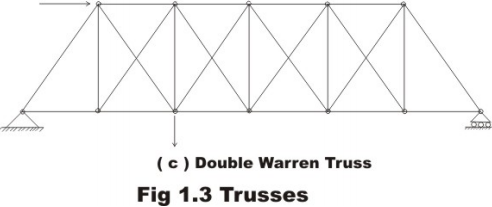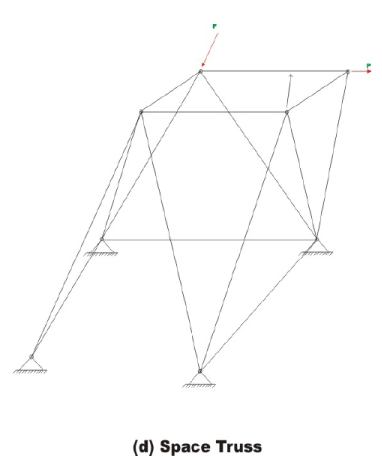Plane frames are also made up of beams and columns, the only difference being they are rigidly connected at the joints as shown in the Fig. 1.4 (a). Major portion of this course is devoted to evaluation of forces in frames for variety of loading conditions. Internal forces at any cross section of the plane frame member are: bending moment, shear force and axial force. As against plane frame, space frames (vide Fig. 1.4 (b)) members may be oriented in any direction. In this case, there is no restriction of how loads are applied on the space frame.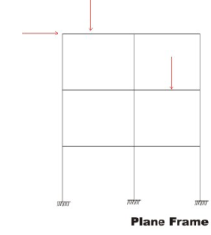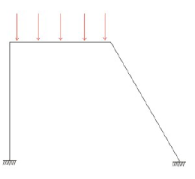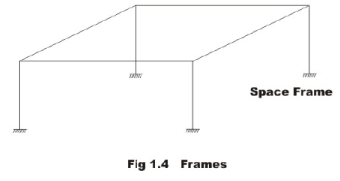Equations of Static Equilibrium

Consider a case where a book is lying on a frictionless table surface. Now, if we apply a force F1 horizontally as shown in the Fig.1.5 (a), then it starts moving in the direction of the force. However, if we apply the force perpendicular to the book as in Fig. 1.5 (b), then book stays in the same position, as in this case the vector sum of all the forces acting on the book is zero. When does an object move and when does it not? This question was answered by Newton when he formulated his famous second law of motion. In a simple vector equation it may be stated as follows: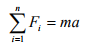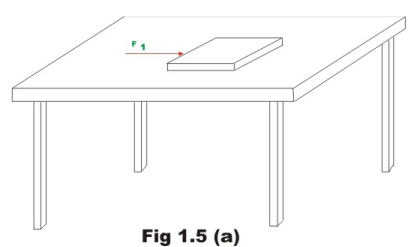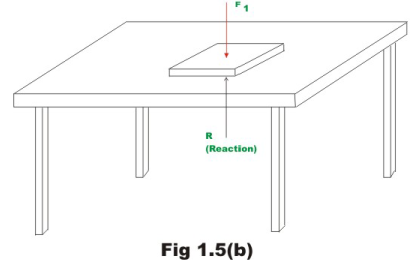where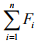is the vector sum of all the external forces acting on the body, m is the total mass of the body and a is the acceleration vector. However, if the body is in the state of static equilibrium then the right hand of equation (1.1) must be zero. Also for a body to be in equilibrium, the vector sum of all external moments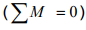about an axis through any point within the body must also vanish. Hence, the book lying on the table subjected to external force as shown in Fig. 1.5 (b) is in static equilibrium. The equations of equilibrium are the direct consequences of Newton’s second law of motion. A vector in 3-dimensions can be resolved into three orthogonal directions viz., x, y and z (Cartesian) coordinate axes. Also, if the resultant force vector is zero then its components in three mutually perpendicular directions also vanish. Hence, the above two equations may also be written in three co-ordinate axes directions as follows: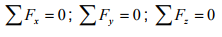(1.2a)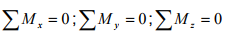(1.2b)

Now, consider planar structures lying in xy - plane. For such structures we could have forces acting only in x and y directions. Also the only external moment that could act on the structure would be the one about the z - axis. For planar structures, the resultant of all forces may be a force, a couple or both. The static equilibrium condition along x -direction requires that there is no net unbalanced force acting along that direction. For such structures we could express equilibrium equations as follows: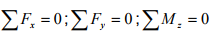(1.3)

Using the above three equations we could find out the reactions at the supports in the beam shown in Fig. 1.6. After evaluating reactions, one could evaluate internal stress resultants in the beam. Admissible or correct solution for reaction and internal stresses must satisfy the equations of static equilibrium for the entire structure. They must also satisfy equilibrium equations for any part of the structure taken as a free body. If the number of unknown reactions is more than the number of equilibrium equations (as in the case of the beam shown in Fig. 1.7), then we can not evaluate reactions with only equilibrium equations. Such structures are known as the statically indeterminate structures. In such cases we need to obtain extra equations (compatibility equations) in addition to equilibrium equations.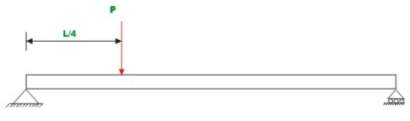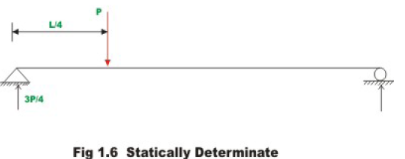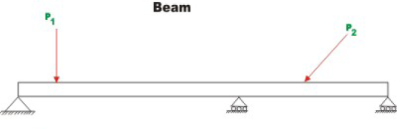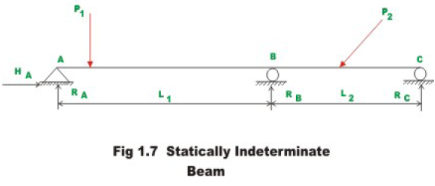Offer running on EduRev: Apply code STAYHOME200 to get INR 200 off on our premium plan EduRev Infinity!

## Structural Analysis

30 videos|122 docs|28 tests

,

,

,

,

,

,

,

,

,

,

,

,

,

,

,

,

,

,

,

,

,

;